# Part 1: Year 10 Quadratic Equations & Non-Linear Simultaneous Equations | Free Worksheet## Part 1: Year 10 Quadratic equations and non-linear simultaneous equations

In this article, we’ll build on what you learned last year when you studied simultaneous equations. Now we’ll explain quadratic equations and non-linear simultaneous equations.

We will step you through the processes for solving different quadratic and non-linear simultaneous equations. Then you can test your skills and knowledge on our concept check questions. This guide provides you with worked examples so you can see where you went wrong, or right!

We will teach you how to solve non-linear simultaneous equations and quadratic equations.

This page will also teach you how to interpret a graph to inspect how many solutions there are to an equation without solving the equation.

## NSW Syllabus Outcomes

Stage 5.3: Solves complex linear, quadratic, simple cubic and simultaneous equations and rearranges literal equations. (MAS.3-7NA)

Solve simultaneous equations, where one equation is non-linear, using algebraic and graphical techniques, including the use of digital technologies

• Use analytical methods to solve a variety of simultaneous equations where one equation is non-linear
1. Choose an appropriate method to solve a pair of simultaneous equations
• Solve pairs of simultaneous equations, where one equation is non-linear, by finding the point of intersection of their graphs using digital technologies.
1. Determine and explain that some pairs of simultaneous equations, where one equation is non-linear, may not have real solutions.

These Outcomes are building on the previous unit in Year 9. Here you will learn to solve equations algebraically and graphically with an $$x^2$$ term. For example, $$y=3x+2$$ and $$x^2+y^2=1$$.

## Assumed Knowledge

Students should be familiar with basic algebraic techniques and simple arithmetic.

Knowledge of basic curve sketching will also be required.

For harder problems, students should also know how to sketch more complicated functions such as hyperbolae, circles, semi-circles, exponential functions and cubic curves – but students will still understand the theory behind the content without knowing how to sketch these harder functions as they may instead use technology to do so.

## Want to see how well you know Quadratic Equations?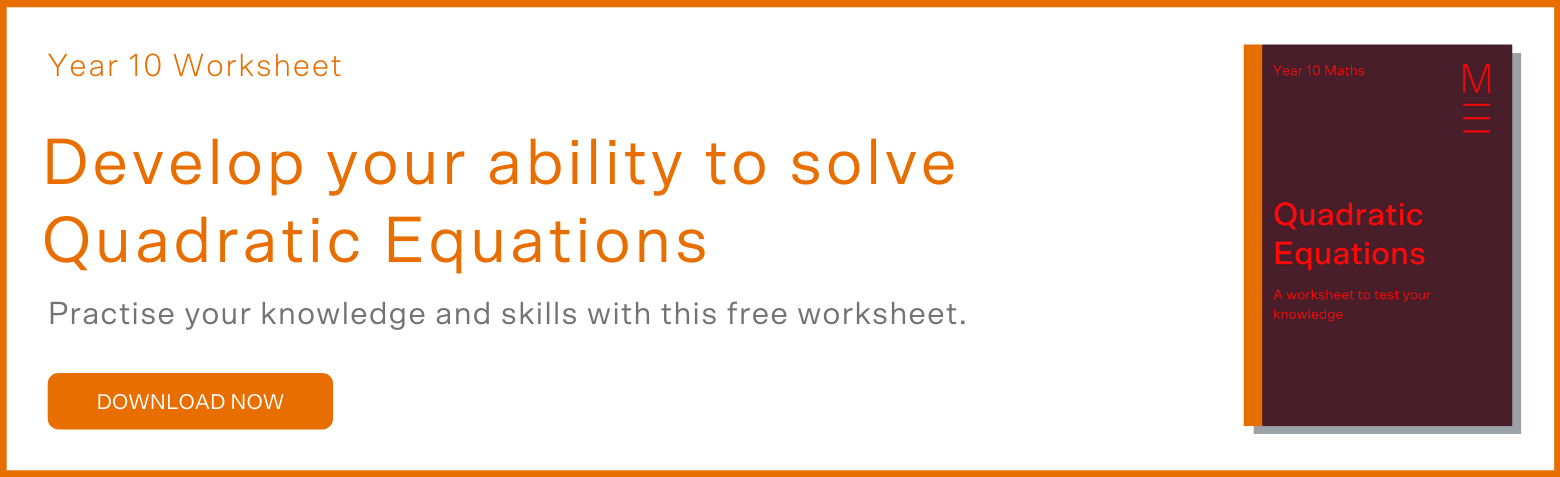## Non-Linear Simultaneous Equations:

We have learnt the graphical, substitution and elimination method for linear sets of equations. Now we can apply the same techniques to solve non-linear equations.

### Example:

1. Solve for the points of intersection of the line $$y=x+1$$ and the circle $$x^2+y^2=4$$.

From sketching a graph, we can see that there are two points of intersection;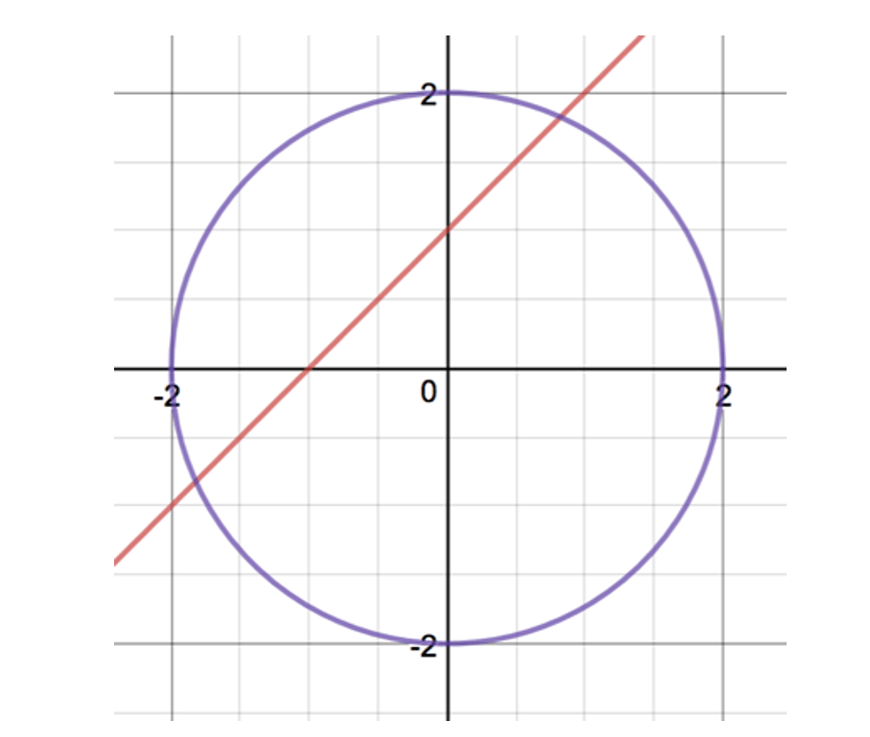But it is not at all obvious what the exact points of intersection are. (Solving this problem does not need a graph).

Elimination method is not so useful here since one equation involves $$x$$ and $$y$$ while the other involves $$x^2$$ and $$y^2$$.

So we will use the substitution method.

$$y=x+1…(1) \\ x^2+y^2=4 …(2) \\$$

Substituting y from (1) into (2), we obtain

$$x^2+(x+1)^2=4 \\ 2x^2+2x-3=0 \\ x=\frac{-2±\sqrt{28}}{4} \text{ using the quadratic formula} \\ =-\frac{1}{2}-\frac{\sqrt{7}}{2} \text{ and } \frac{\sqrt{7}}{2}-\frac{1}{2} \\$$

Substitute these $$x$$ values back into either (1) or (2) (we immediately see that (1) is easier) to solve for the corresponding value for $$y$$.

When $$x=-\frac{1}{2}-\frac{\sqrt{7}}{2} ,y=\frac{1}{2}-\frac{\sqrt{7}}{2}$$ and when $$x=\frac{\sqrt{7}}{2}-\frac{1}{2},y=\frac{1}{2}+\frac{\sqrt{7}}{2}$$

and thus $$(-\frac{1}{2}- \frac{\sqrt7}{2}, \frac{1}{2}-\frac{\sqrt7}{2})$$ and $$(\frac{{\sqrt7}}{2}-\frac{1}{2},\frac{1}{2}+\frac{\sqrt7}{2})$$ are the points of intersection.

## Analysing the Number of Real Solutions of an Equation or Pair of Simultaneous Equations.

It was said earlier that “the solution to a set of simultaneous equations is the common point of intersection”.

This observation makes it very easy to find the number of solutions to an equation without having to solve it.

The number of solutions to the equation

$$ax+b=cx+d$$

or the pair of equations:

$$y=ax+b$$ and  $$y=cx+d$$

is the number of points of intersection caused by graphing $$y=ax+b$$ and $$y=cx+d$$ on the same number plane.

In general, for students who have seen functional notation, the number of solutions to an equation

$$f(x)=g(x)$$

is simply the number of points of intersection caused by graphing $$y=f(x)$$ and $$y=g(x)$$ on the same number plane.

### Example 1 :

How many solutions are there to the set of simultaneous equations:

$$2x+y-20=0$$ and $$6x+3y=1$$

### Solution 1 :

Since both lines have the same gradient, they are parallel.

Parallel lines never intersect so there will be no solutions.

Graphing the two lines, we can see this visually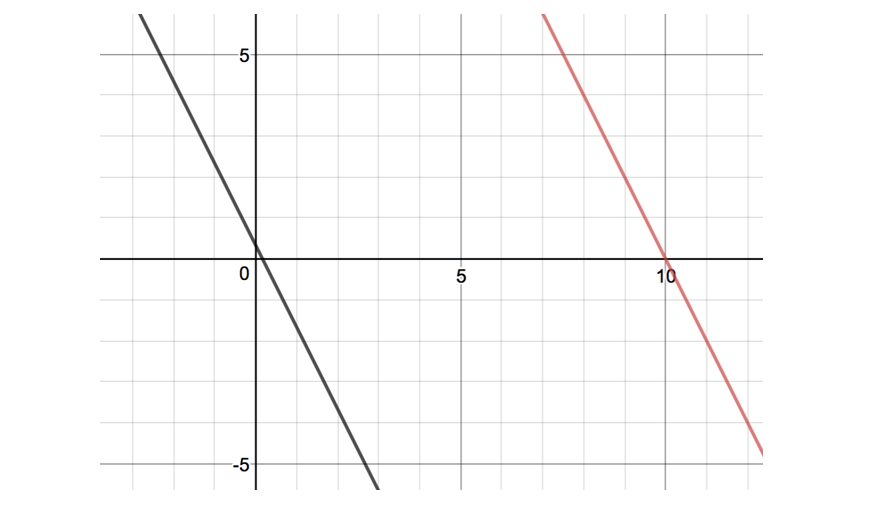### Example 2 :

Using technology, how many solutions are there to the simultaneous equations

$$y^2=4x$$ and $$x^3+y^3=100$$

The graphing package Desmos gives the following graph: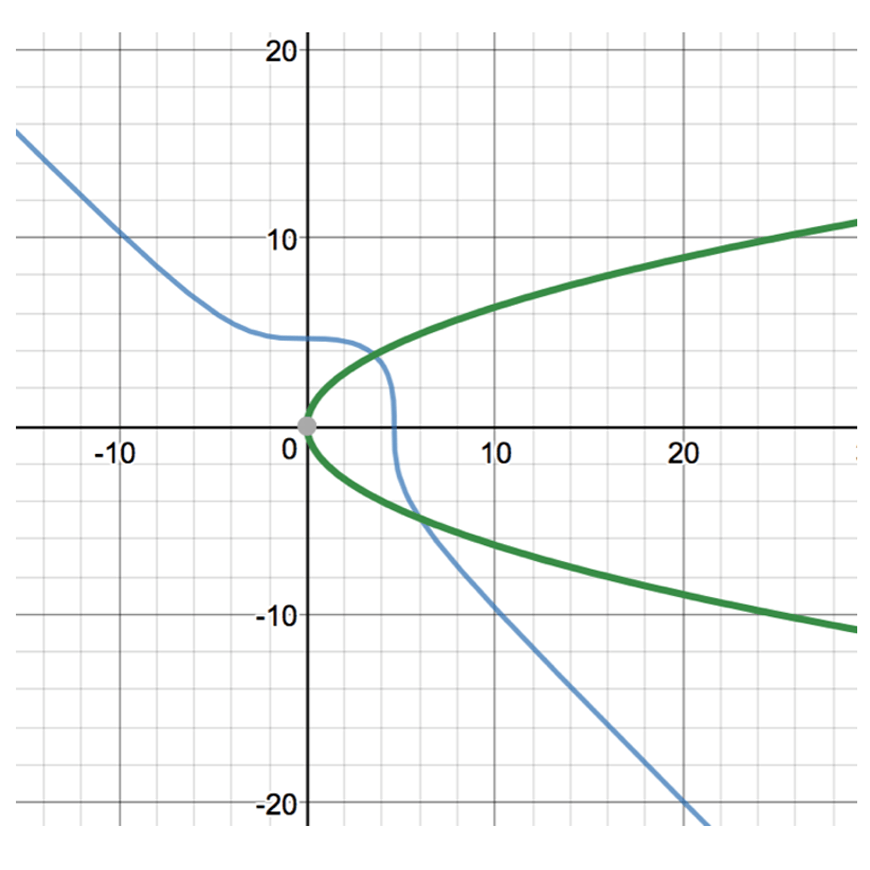Counting the points of intersection, we can see that there are 2 solutions.

## Summary

• If a point $$P(x,y)$$ satisfies two equations. Then $$x$$ and $$y$$ is a solution to the pair of simultaneous equations.
• Solving two equations simultaneously is equivalent to solving for the point of intersection of two graphs.

### Graphical Method

• Sketch each equation as a function, and graphically see where the solution is.
• Will not always be helpful since it can be hard to sketch accurately enough to see what the exact values of the solutions are.

### Substitution Method

• Substitute one of the variables in equation 1 into equation 2. Then, turn the problem into solving a linear equation.

### Elimination Method

• By adding/subtracting the equations, you eliminate one of the variables. Now, you can solve for the other variable by solving a linear equation.
• Sometimes you will need to multiply equations by a constant in order to eliminate.

### Number of Solutions

• When you sketch the LHS and RHS as graphs on the same number plane, the number of points of intersections is the number of solutions to an equation

## Concept Check Questions:

1. Solve for the points of intersection that the line $$y=2x+1$$ makes with the hyperbola $$y=\frac{1}{x}$$

2. Solve for the points of intersection that the line $$y=x-1$$ makes with the circle  $$x^2+y^2=1$$

3. By sketching the two curves, explain why $$x^2=x-1$$ has no solutions

4. By sketching the two lines, explain why the pair of equations $$x+2y=1$$ and $$2x+4y=5$$ have no solution when solved simultaneously.

5. By using technology or your knowledge of curve sketching, sketch $$y=\frac{1}{2} x^2$$ and $$x^2+y^2=1$$ on the same number plane and hence state the number of solutions for the equation $$x^2+\frac{1}{4} x^4=1$$

6. It is actually possible to solve more than two equations simultaneously.

The general equation of a parabola is $$y=ax^2+bx+c$$.

Given that a particular parabola passes through the points $$(1,2), (-1,0)$$ and $$(2,9)$$, show that this results in the three equations:

$$a+b+c=2…(1) \\ a-b+c=0…(2) \\ 4a+2b+c=9…(3) \\$$

7. CHALLENGE

Use a clever elimination by considering (a) and (b) to solve for $$b$$ immediately in Question 9 above. Then solve for $$a$$ and $$c$$ in order to give the equation of the parabola in Question 9.

## Solutions

1.

$$y= \frac{1}{x}…(1) \\ y=2x+1…(2) \\$$

Substitute (1) into (2):

$$\frac{1}{x}=2x+1 \\ 2x^2+x-1=0 \\ (x+1)(2x-1)=0 \\ x=-1, \frac{1}{2} \\$$

When $$x=-1, y=-1$$ and when $$x= \frac{1}{2}, y=2$$

Thus $$(-1,-1)$$ and $$(\frac{1}{2},2)$$ are the points of intersection.

2.

$$y=x-1…(1) \\ x^2+y^2=1 …(2) \\$$

Substitute for $$y$$ from (1) into (2):

$$x^2+(x-1)^2=1 \\ 2x^2-2x+1=1 \\ x(x-1)=0 \\ x=0,1 \\$$

When $$x=0,y=-1$$ and when $$x=1,y=0$$

Thus $$(0,-1)$$ and $$(1,0)$$ are the points of intersection

3.

We first sketch $$y=x^2$$ and $$y=x-1$$ on the same number plane: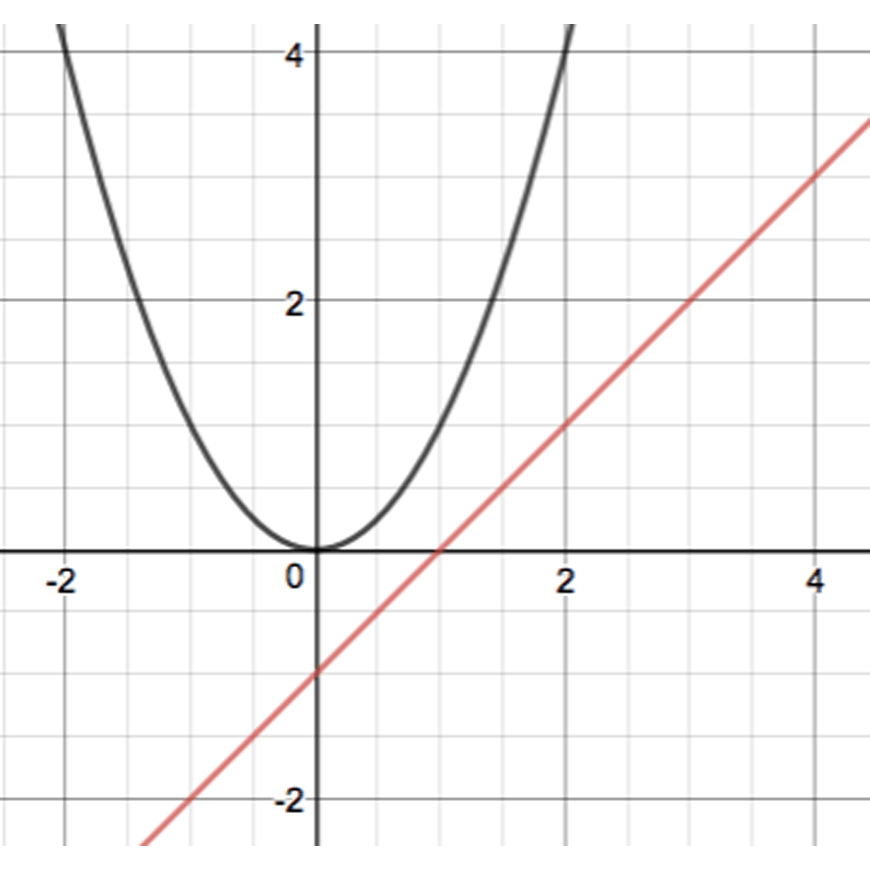There are no points of intersection, thus there are no solutions.

4.

By sketching the two lines, explain why the pair of equations $$x+2y=1$$ and $$2x+4y=5$$ have no solution when solved simultaneously.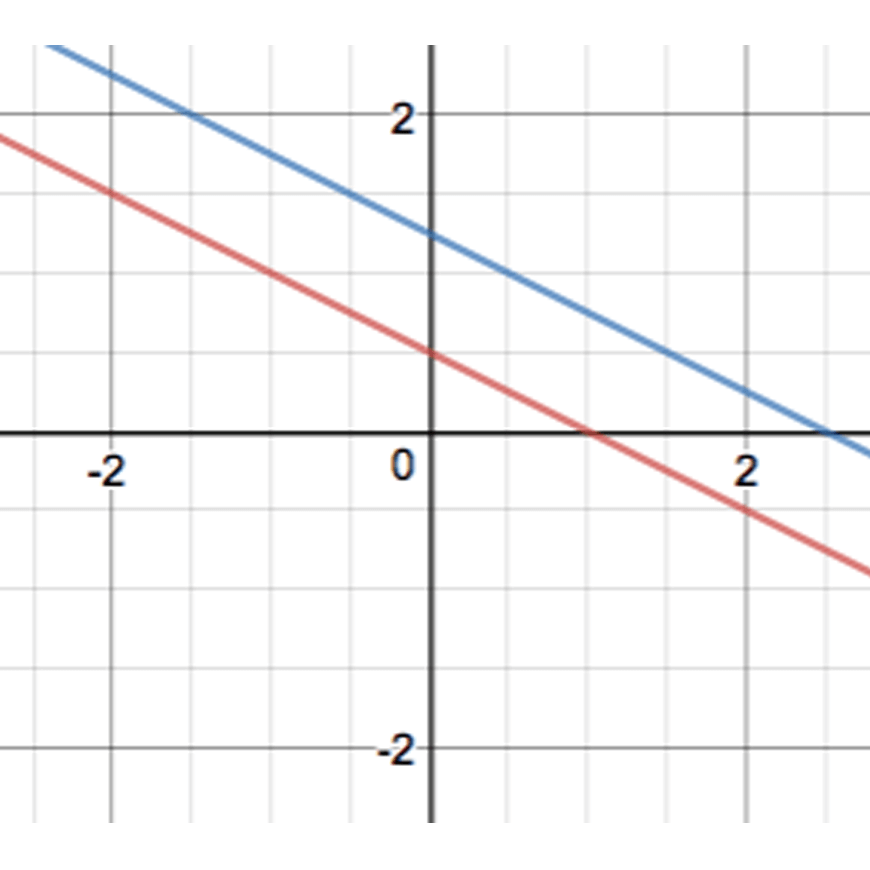The two lines are parallel and thus will never intersect. So the equations will have no solution when solved simultaneously.

5.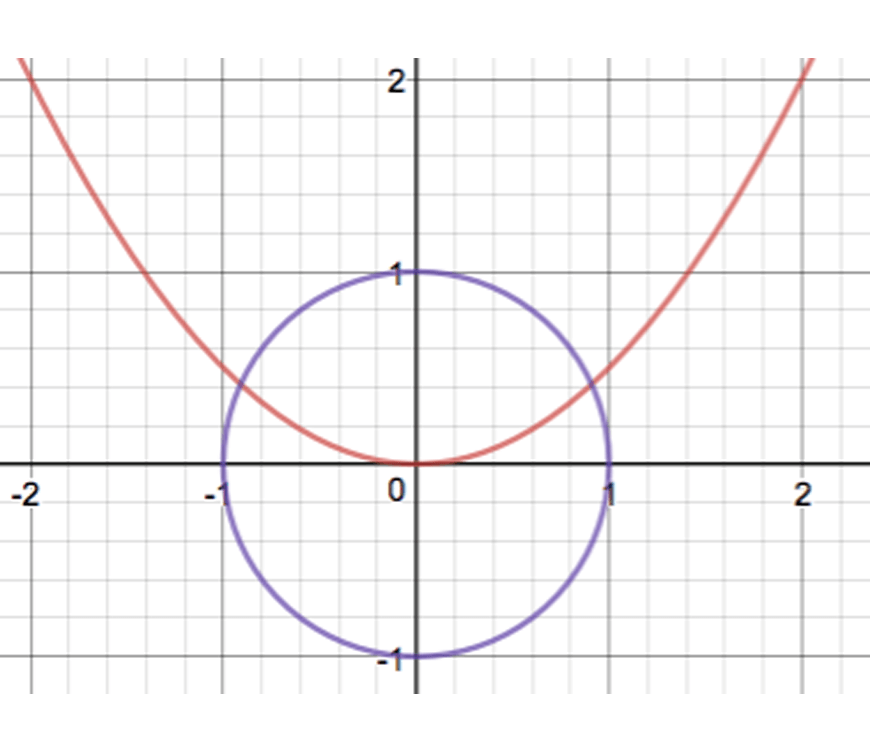We see that there are two solutions to the pair of simultaneous equations. Substituting the parabola into the circle, we get that

$$x^2+(\frac{1}{2} x^2 )^2=1 \\ x^2+\frac{1}{4} x^4=1 \\$$

And so this equation will have two solutions.

6.

Since the parabola passes through the three points, we should be able to substitute each point into the general equation of the parabola to obtain the three equations.

$$(1,2)⇒2=a×1^2+b×1+c \\ (-1,0)⇒0=a×(-1)^2+b×(-1)+c \\ (2,9)⇒9=a×2^2+b×2+c \\$$

which simplifies to the three equations needed.

7.

$$a+b+c=2…(1) \\ a-b+c=0…(2) \\ 4a+2b+c=9…(3) \\ \\ (1)-(2): 2b=2⇒b=1\\$$

Now we can rewrite (1),(2) and (3)

$$a+c=1 …(1) \\ a+c=1 …(2) \\ 4a+c=7 …(3) \\$$

(1) and (2) give us the same information, so we now need to use (3) as well.

(3)-(1) gives

$$3a=6⇒a=2$$

So (1) can now be solved for c:

$$2+c=1⇒c=-1$$

Hence $$a=2,b=1,c=-1$$

Thus the parabola that passes through the points $$(1,2),(-1,0)$$ and $$(2,9)$$ is

$$y=2x^2+x-1$$

## Additional Challenging Questions (From the MAX Series)

### Questions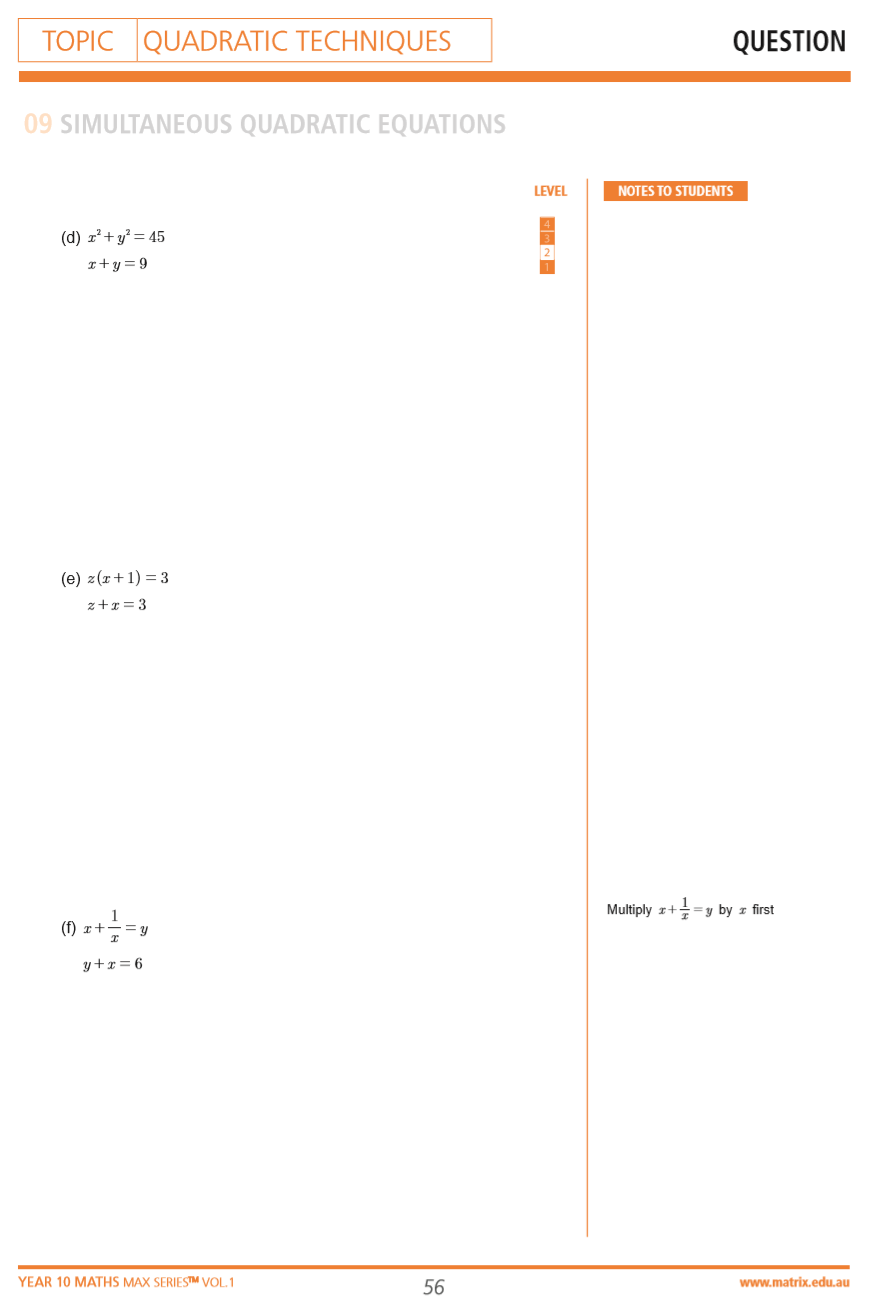Questions from the Year 10 MAX Series Vol. 1 Quadratic Techniques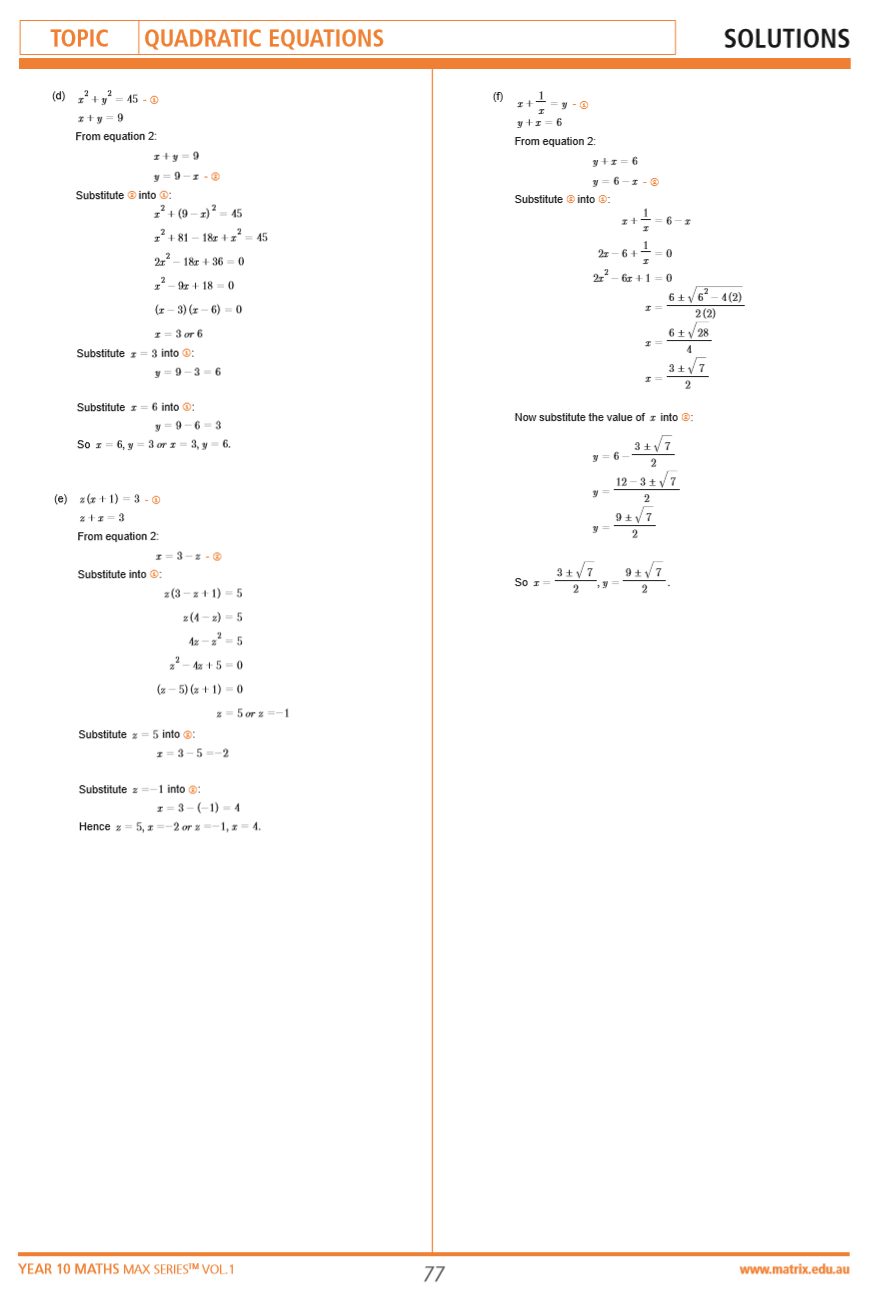Solutions from the Year 10 MAX Series Vol. 1 Quadratic Techniques

## Want to quickly revise your term’s content and receive immediate feedback?

In our 2-Day Year 10 Maths Holiday Course, we will teach you key Maths concepts and provide you with questions to practice!## Feeling improbable about scoring high in probability?

In our next article, we discuss probability. Read on if you want to increase your odds of acing Year 10 Maths!

© Matrix Education and www.matrix.edu.au, 2021. Unauthorised use and/or duplication of this material without express and written permission from this site’s author and/or owner is strictly prohibited. Excerpts and links may be used, provided that full and clear credit is given to Matrix Education and www.matrix.edu.au with appropriate and specific direction to the original content.